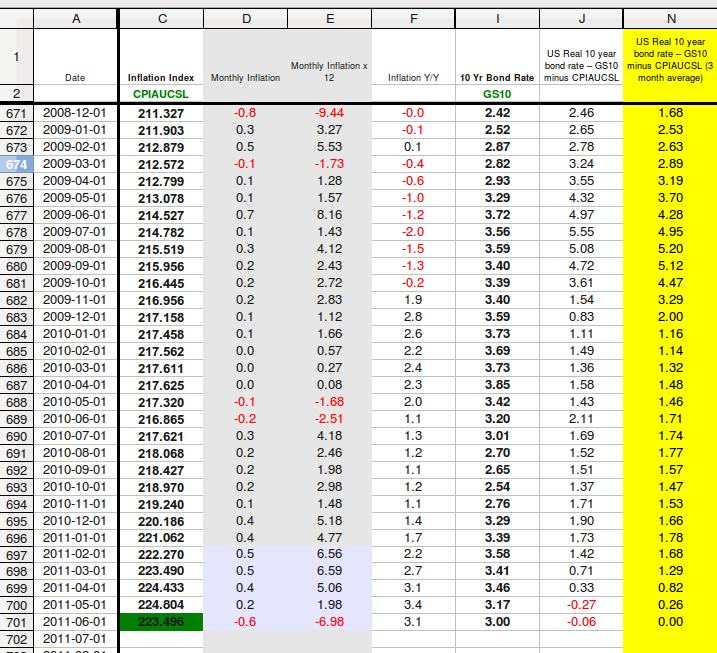## 2011-07-07

### The chance of avoiding another downturn is now almost impossible

10 Year bond rates for June 2011 came in at 3.00%. Inflation figures for the same month are due on 2011-07-15 (Friday next week).

In order for the US Real 10 year bond rate to remain positive, June inflation needs to have an index reading of 223.496 - which implies a monthly deflationary result of -0.6%. At this point there is very little evidence of a deflationary hit in June (eg soaring US dollar, credit crunch, large drop in sharemarket value).

Take a look at my spreadsheet.June's potential recession avoiding index result of 223.496 is shown there in green. Any inflation index result of 223.497 or higher will result in column N (real 10 year bond rates averaged over three months) moving into negative. This presages a recession. When the inflation data comes out Friday next week, a coming downturn will be confirmed.

For those of you who are spreadsheet minded, here are the details:
• Column C data from here.
• Column D equation (at 701) is =PRODUCT(((C701-C700)/C700)*100).
• Column E equation (at 701) is =PRODUCT(D701*12).
• Column F equation (at 701) is =PRODUCT(((C701-C689)/C689)*100). (This is the "headline inflation" result).
• Column I data from here.
• Column J equation (at 701) is =SUM(I701-F701).
• Column N equation (at 701) is =AVERAGE(J699:J701).